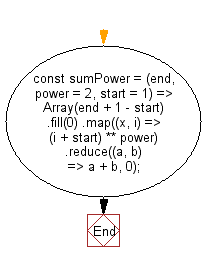# JavaScript: Get the sum of the powers of all the numbers from start to end

## JavaScript fundamental (ES6 Syntax): Exercise-135 with Solution

Write a JavaScript program to get the sum of the powers of all the numbers from start to end (both inclusive).

• Use Array.prototype.fill() to create an array of all the numbers in the target range.
• Use Array.prototype.map() and the exponent operator (**) to raise them to power and Array.prototype.reduce() to add them together.
• Omit the second argument, power, to use a default power of 2.
• Omit the third argument, start, to use a default starting value of 1.

Sample Solution:

JavaScript Code:

``````//#Source https://bit.ly/2neWfJ2
const sumPower = (end, power = 2, start = 1) =>
Array(end + 1 - start)
.fill(0)
.map((x, i) => (i + start) ** power)
.reduce((a, b) => a + b, 0);

console.log(sumPower(10));
console.log(sumPower(10, 3));
console.log(sumPower(10, 3, 5));
```
```

Sample Output:

```385
3025
2925
```

Pictorial Presentation:Flowchart:Live Demo:

See the Pen javascript-basic-exercise-135-1 by w3resource (@w3resource) on CodePen.

Improve this sample solution and post your code through Disqus

What is the difficulty level of this exercise?

Test your Programming skills with w3resource's quiz.

﻿

## JavaScript: Tips of the Day

Chunks an array into n smaller arrays

Example:

```const tips_chunkIntoN = (arr, n) => {
const size = Math.ceil(arr.length / n);
return Array.from({ length: n }, (v, i) =>
arr.slice(i * size, i * size + size)
);
}
console.log(tips_chunkIntoN([1, 2, 3, 4, 5, 6, 7,8], 4));
```

Output:

```[[1,2],[3,4],[5,6],[7,8]]
```